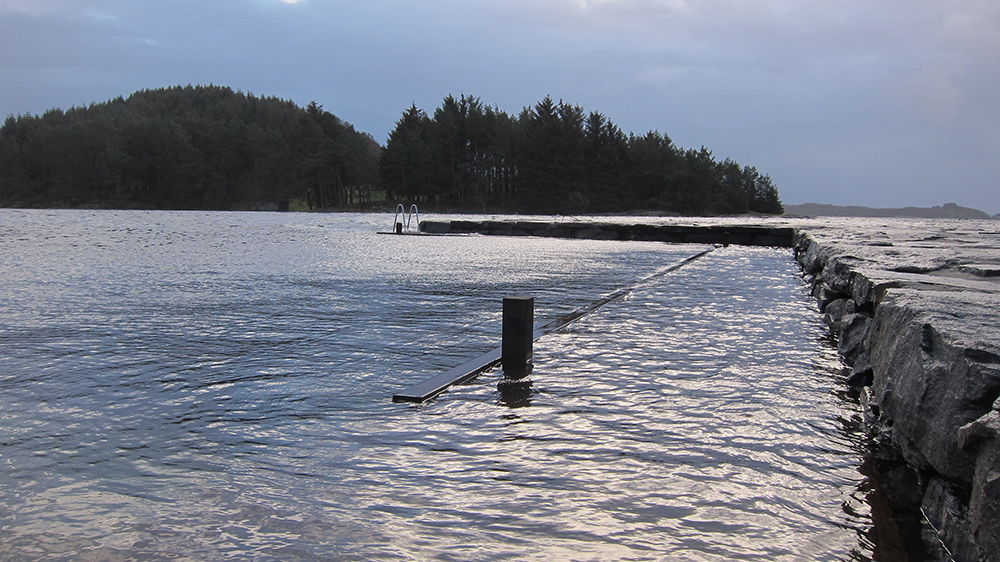HIGH WATER LEVEL: The ideal pier height depends on the size of the boats that are to be moored there, and whether the pier can withstand being submerged underwater during periods of extra high water levels. In addition, the tidal differences are crucial. Photo: Bente Berggraf
Last updated

# How High Does a Pier Need to Be?

Piers that are too low become submerged underwater at high tide. If the pier is too high, it might be difficult to exit your boat. This method will help you find the ideal height for your pier.

The ideal pier height depends on the size of the boats that are to be moored there, and whether the pier can withstand being submerged underwater during periods of extra high water levels. In addition, the tidal differences are crucial.

## Applications

The method below can be used when calculating any heights along the shoreline, and can also be used to find the ideal height when building a boathouse.

It is important to note that the method will not provide exact measurements for areas that are far from our permanent tide gauges. However, for most practical purposes, the result is good enough.

It is also important to note that wave heights will come in addition to measured water levels, therefor wave heights must also be taken into account. The Norwegian Mapping Authority does not measure wave heights, but both the Norwegian Meteorological Institute and the Norwegian Coastal Administration issue wave forecasts.

#### Fact boxDefinitions

Chart Datum: Zero level on nautical charts. Depths on nautical charts have Chart Datum as the reference level. Chart Datum is so low that the actual water level rarely gets below this level, ensuring that boats do not run aground.

Tides: Changes in water level caused by variations in gravitational forces from the moon and the sun.

Water level: The height of the water surface in one particular place at a given time. Oceanic water levels are affected by tides and the weather (wind, air pressure, etc.).

## Method for determining pier height

This is an example from Smøla.

### 1. Time

Choose a time when the sea is calm.

### 2. Measure the distance

Measure the distance from the deck of the pier down to the surface of the water. Make a note of the exact time you take the measurement.

An example from Smøla: On 8 April, 2020 at 10:00 am, the distance from the deck of the pier to the surface of the water was 105 cm.

### 3. Conduct a location search on Se havnivå

Visit the Se havnivå online service and search for the place/location where you measured the distance.

• Make sure that the reference level box for Chart Datum (zero level on nautical charts) is ticked.
• Select the date when you measured the distance (from the surface of the water to the deck of the pier).
• Find out (and make a note of) what the water level was when you measured:
• Navigate the mouse cursor along the blue curve in the graph which shows the observed water level.
• Alternatively, you can choose the table view and 10-minute interval.

An example from Smøla: Wednesday, 8 April, 2020 at 10:00 am, the water level at Smøla was 198 cm above Chart Datum.

### 4. Calculate

The height of the pier in relation to Chart Datum
= Water level above chart datum + measured value (deck of pier above surface of water)

An example from Smøla: 198 cm + 105 cm = 303 cm

We have measured that the deck of the pier is 105 cm higher than the surface of the water. When we add the value of the measured water level (198 cm), the calculation shows that the deck of the pier is 303 cm above Chart Datum.

### 5. Compare height of pier with different vertical water levels

Then, compare ‘the height of the pier in relation to Chart Datum’ with the figure of water levels for the place you have measured. Make sure that the reference level of the figure is Chart Datum.

You can then find out how high the deck of the pier is in relation to different water levels, such as the water level for ‘High water with 5 year return period’.

An example from Smøla: Based on the figure of levels for Smøla, we see that 303 cm above  Chart Datum corresponds approximately to the level of high water with 5 year return period, 302 cm. In statistical terms, this means that on average, the water level will reach the deck of the pier once every five years.

## Things to consider:

• The pier should have a suitable height so that everyone can exit a boat when going ashore. Therefore, you might want to consider building a pier that can withstand being submerged underwater during certain periods.
• How big are the boats that are most likely to moor at the pier?
• How big is the tidal difference?
• Will the boat run aground at low tide?
• Take wave height into account, as this is comes addition to measured water level.
Share